# sklearn.compose.ColumnTransformer¶

class sklearn.compose.ColumnTransformer(transformers, *, remainder='drop', sparse_threshold=0.3, n_jobs=None, transformer_weights=None, verbose=False, verbose_feature_names_out=True)[source]

Applies transformers to columns of an array or pandas DataFrame.

This estimator allows different columns or column subsets of the input to be transformed separately and the features generated by each transformer will be concatenated to form a single feature space. This is useful for heterogeneous or columnar data, to combine several feature extraction mechanisms or transformations into a single transformer.

Read more in the User Guide.

New in version 0.20.

Parameters:
transformerslist of tuples

List of (name, transformer, columns) tuples specifying the transformer objects to be applied to subsets of the data.

namestr

Like in Pipeline and FeatureUnion, this allows the transformer and its parameters to be set using set_params and searched in grid search.

transformer{‘drop’, ‘passthrough’} or estimator

Estimator must support fit and transform. Special-cased strings ‘drop’ and ‘passthrough’ are accepted as well, to indicate to drop the columns or to pass them through untransformed, respectively.

columnsstr, array-like of str, int, array-like of int, array-like of bool, slice or callable

Indexes the data on its second axis. Integers are interpreted as positional columns, while strings can reference DataFrame columns by name. A scalar string or int should be used where transformer expects X to be a 1d array-like (vector), otherwise a 2d array will be passed to the transformer. A callable is passed the input data X and can return any of the above. To select multiple columns by name or dtype, you can use make_column_selector.

remainder{‘drop’, ‘passthrough’} or estimator, default=’drop’

By default, only the specified columns in transformers are transformed and combined in the output, and the non-specified columns are dropped. (default of 'drop'). By specifying remainder='passthrough', all remaining columns that were not specified in transformers, but present in the data passed to fit will be automatically passed through. This subset of columns is concatenated with the output of the transformers. For dataframes, extra columns not seen during fit will be excluded from the output of transform. By setting remainder to be an estimator, the remaining non-specified columns will use the remainder estimator. The estimator must support fit and transform. Note that using this feature requires that the DataFrame columns input at fit and transform have identical order.

sparse_thresholdfloat, default=0.3

If the output of the different transformers contains sparse matrices, these will be stacked as a sparse matrix if the overall density is lower than this value. Use sparse_threshold=0 to always return dense. When the transformed output consists of all dense data, the stacked result will be dense, and this keyword will be ignored.

n_jobsint, default=None

Number of jobs to run in parallel. None means 1 unless in a joblib.parallel_backend context. -1 means using all processors. See Glossary for more details.

transformer_weightsdict, default=None

Multiplicative weights for features per transformer. The output of the transformer is multiplied by these weights. Keys are transformer names, values the weights.

verbosebool, default=False

If True, the time elapsed while fitting each transformer will be printed as it is completed.

verbose_feature_names_outbool, default=True

If True, get_feature_names_out will prefix all feature names with the name of the transformer that generated that feature. If False, get_feature_names_out will not prefix any feature names and will error if feature names are not unique.

New in version 1.0.

Attributes:
transformers_list

The collection of fitted transformers as tuples of (name, fitted_transformer, column). fitted_transformer can be an estimator, ‘drop’, or ‘passthrough’. In case there were no columns selected, this will be the unfitted transformer. If there are remaining columns, the final element is a tuple of the form: (‘remainder’, transformer, remaining_columns) corresponding to the remainder parameter. If there are remaining columns, then len(transformers_)==len(transformers)+1, otherwise len(transformers_)==len(transformers).

named_transformers_Bunch

Access the fitted transformer by name.

sparse_output_bool

Boolean flag indicating whether the output of transform is a sparse matrix or a dense numpy array, which depends on the output of the individual transformers and the sparse_threshold keyword.

output_indices_dict

A dictionary from each transformer name to a slice, where the slice corresponds to indices in the transformed output. This is useful to inspect which transformer is responsible for which transformed feature(s).

New in version 1.0.

n_features_in_int

Number of features seen during fit. Only defined if the underlying transformers expose such an attribute when fit.

New in version 0.24.

make_column_transformer

Convenience function for combining the outputs of multiple transformer objects applied to column subsets of the original feature space.

make_column_selector

Convenience function for selecting columns based on datatype or the columns name with a regex pattern.

Notes

The order of the columns in the transformed feature matrix follows the order of how the columns are specified in the transformers list. Columns of the original feature matrix that are not specified are dropped from the resulting transformed feature matrix, unless specified in the passthrough keyword. Those columns specified with passthrough are added at the right to the output of the transformers.

Examples

>>> import numpy as np
>>> from sklearn.compose import ColumnTransformer
>>> from sklearn.preprocessing import Normalizer
>>> ct = ColumnTransformer(
...     [("norm1", Normalizer(norm='l1'), [0, 1]),
...      ("norm2", Normalizer(norm='l1'), slice(2, 4))])
>>> X = np.array([[0., 1., 2., 2.],
...               [1., 1., 0., 1.]])
>>> # Normalizer scales each row of X to unit norm. A separate scaling
>>> # is applied for the two first and two last elements of each
>>> # row independently.
>>> ct.fit_transform(X)
array([[0. , 1. , 0.5, 0.5],
[0.5, 0.5, 0. , 1. ]])


ColumnTransformer can be configured with a transformer that requires a 1d array by setting the column to a string:

>>> from sklearn.feature_extraction import FeatureHasher
>>> from sklearn.preprocessing import MinMaxScaler
>>> import pandas as pd
>>> X = pd.DataFrame({
...     "documents": ["First item", "second one here", "Is this the last?"],
...     "width": [3, 4, 5],
... })
>>> # "documents" is a string which configures ColumnTransformer to
>>> # pass the documents column as a 1d array to the FeatureHasher
>>> ct = ColumnTransformer(
...     [("text_preprocess", FeatureHasher(input_type="string"), "documents"),
...      ("num_preprocess", MinMaxScaler(), ["width"])])
>>> X_trans = ct.fit_transform(X)


Methods

 fit(X[, y]) Fit all transformers using X. fit_transform(X[, y]) Fit all transformers, transform the data and concatenate results. get_feature_names_out([input_features]) Get output feature names for transformation. get_params([deep]) Get parameters for this estimator. set_output(*[, transform]) Set the output container when "transform" and "fit_transform" are called. set_params(**kwargs) Set the parameters of this estimator. Transform X separately by each transformer, concatenate results.
fit(X, y=None)[source]

Fit all transformers using X.

Parameters:
X{array-like, dataframe} of shape (n_samples, n_features)

Input data, of which specified subsets are used to fit the transformers.

yarray-like of shape (n_samples,…), default=None

Targets for supervised learning.

Returns:
selfColumnTransformer

This estimator.

fit_transform(X, y=None)[source]

Fit all transformers, transform the data and concatenate results.

Parameters:
X{array-like, dataframe} of shape (n_samples, n_features)

Input data, of which specified subsets are used to fit the transformers.

yarray-like of shape (n_samples,), default=None

Targets for supervised learning.

Returns:
X_t{array-like, sparse matrix} of shape (n_samples, sum_n_components)

Horizontally stacked results of transformers. sum_n_components is the sum of n_components (output dimension) over transformers. If any result is a sparse matrix, everything will be converted to sparse matrices.

get_feature_names_out(input_features=None)[source]

Get output feature names for transformation.

Parameters:
input_featuresarray-like of str or None, default=None

Input features.

• If input_features is None, then feature_names_in_ is used as feature names in. If feature_names_in_ is not defined, then the following input feature names are generated: ["x0", "x1", ..., "x(n_features_in_ - 1)"].

• If input_features is an array-like, then input_features must match feature_names_in_ if feature_names_in_ is defined.

Returns:
feature_names_outndarray of str objects

Transformed feature names.

get_params(deep=True)[source]

Get parameters for this estimator.

Returns the parameters given in the constructor as well as the estimators contained within the transformers of the ColumnTransformer.

Parameters:
deepbool, default=True

If True, will return the parameters for this estimator and contained subobjects that are estimators.

Returns:
paramsdict

Parameter names mapped to their values.

property named_transformers_

Access the fitted transformer by name.

Read-only attribute to access any transformer by given name. Keys are transformer names and values are the fitted transformer objects.

set_output(*, transform=None)[source]

Set the output container when "transform" and "fit_transform" are called.

Calling set_output will set the output of all estimators in transformers and transformers_.

Parameters:
transform{“default”, “pandas”}, default=None

Configure output of transform and fit_transform.

• "default": Default output format of a transformer

• "pandas": DataFrame output

• None: Transform configuration is unchanged

Returns:
selfestimator instance

Estimator instance.

set_params(**kwargs)[source]

Set the parameters of this estimator.

Valid parameter keys can be listed with get_params(). Note that you can directly set the parameters of the estimators contained in transformers of ColumnTransformer.

Parameters:
**kwargsdict

Estimator parameters.

Returns:
selfColumnTransformer

This estimator.

transform(X)[source]

Transform X separately by each transformer, concatenate results.

Parameters:
X{array-like, dataframe} of shape (n_samples, n_features)

The data to be transformed by subset.

Returns:
X_t{array-like, sparse matrix} of shape (n_samples, sum_n_components)

Horizontally stacked results of transformers. sum_n_components is the sum of n_components (output dimension) over transformers. If any result is a sparse matrix, everything will be converted to sparse matrices.

## Examples using sklearn.compose.ColumnTransformer¶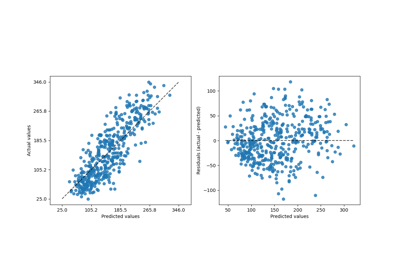Release Highlights for scikit-learn 1.2

Release Highlights for scikit-learn 1.2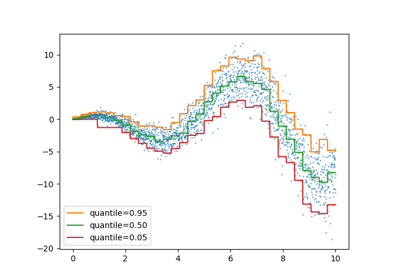Release Highlights for scikit-learn 1.1

Release Highlights for scikit-learn 1.1Release Highlights for scikit-learn 1.0

Release Highlights for scikit-learn 1.0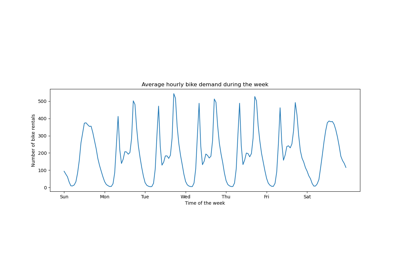Time-related feature engineering

Time-related feature engineering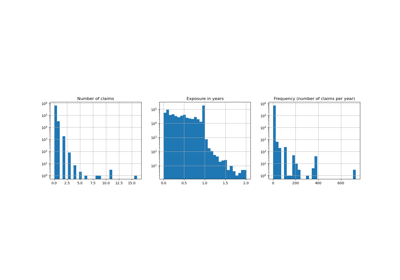Poisson regression and non-normal loss

Poisson regression and non-normal loss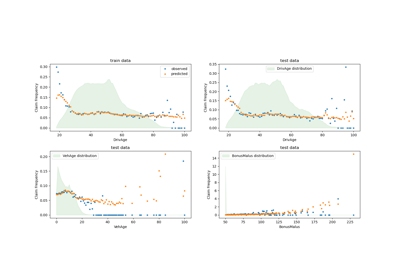Tweedie regression on insurance claims

Tweedie regression on insurance claims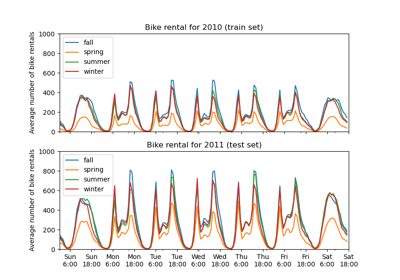Partial Dependence and Individual Conditional Expectation Plots

Partial Dependence and Individual Conditional Expectation Plots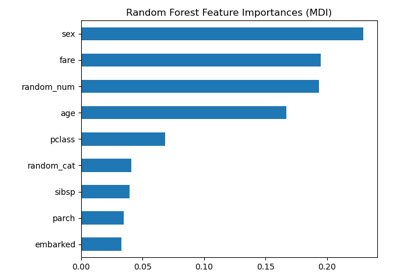Permutation Importance vs Random Forest Feature Importance (MDI)

Permutation Importance vs Random Forest Feature Importance (MDI)Displaying Pipelines

Displaying Pipelines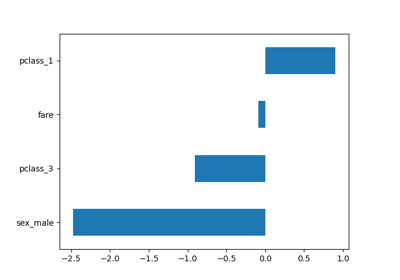Introducing the set_output API

Introducing the set_output APIColumn Transformer with Heterogeneous Data Sources

Column Transformer with Heterogeneous Data SourcesColumn Transformer with Mixed Types

Column Transformer with Mixed Types